# 神经网络变得轻松（第十一部分）：自 GPT 获取

26 四月 2021, 13:44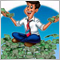0
1 224

### 2. GPT 与之前研究的变换器之间的区别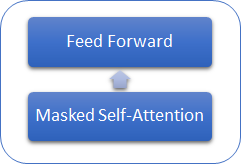### 3. 实现

1. 令牌的输入序列会被馈入到变换器模块之中。
2. 针对所有自关注目击者的一个序列。 进而，对于每个关注的目击者，2-5 中的动作是相同的。

3. 令牌向量乘以相应的权重矩阵 W（已训练），可计算每个令牌的三个向量（query，key，value）。

4. 将 'query' 和 'key' 相乘，我们可判定序列元素之间的依赖性。 在此步骤，将序列中每个元素的向量 'query' 乘以序列中当前元素和所有先前元素的 'key' 向量。

5. 在每个 query 的上下文中，使用 SoftMax 函数对获得的关注得分矩阵进行常规化。 序列的后续元素则设置了零关注分数。
6. 作为第 3 步和第 4 步的结果，我们获得了平方矩阵 Score，该平方矩阵的大小依据序列中元素的数量来确定，在其内每个 'query' 的上下文中所有元素的合计为 “1”。

7. 将常规化的关注分数乘以序列相应元素的 'value' 向量，然后与结果向量相加，我们可以得到序列 (Z) 的每个元素的关注校正值。

8. 接下来，我们基于所有关注目击者的结果断定加权 Z 向量。 为此，将来自所有关注目击者的校正后的 “value” 向量串联到单一向量，然后乘以正在训练的 W0 矩阵。

9. 所得张量会被添加到输入序列，并进行常规化。

10. 多目击者自关注机制后随前馈模块的两个完全连接层。 第一层（隐藏）包含的神经元数量比之含有 ReLU 激活函数的输入序列多 4 倍。 第二层的尺寸等于输入序列的尺寸，且神经元不使用激活函数。

11. 完全连接层的结果与张量求和，其张量将被馈入前馈模块。 然后将生成的张量常规化。

#### 3.1. 为我们的模型创建新类。

 iLayers 模型中的变换器模块数量 iHeads 自关注目击者的数量 iWindow 输入窗口大小（1 个输入序列令牌） iWindowKey 内部向量 Query、Key、Value 的维度 iUnits 输入序列中的元素（令牌）数量

 QKV_Tensors 存储张量 Query、Key、Value，及其梯度的数组 QKV_Weights 存储 Wq、Wk、Wv 权重矩阵、及其动量矩阵集合的数组 S_Tensors 存储 Score 矩阵、及其梯度集合的数组 AO_Tensors 存储自关注机制输出张量、及其梯度的数组 FF_Tensors 存储前馈模块输入、隐藏和输出张量、及其梯度的数组 FF_Weights 存储前馈模块权重矩阵、及其动量的数组。

```class CNeuronMLMHAttentionOCL       :  public CNeuronBaseOCL
{
protected:
uint              iLayers;                                     ///< Number of inner layers
uint              iWindow;                                     ///< Input window size
uint              iUnits;                                      ///< Number of units
uint              iWindowKey;                                  ///< Size of Key/Query window
//---
CCollection       *QKV_Tensors;                                ///< The collection of tensors of Queries, Keys and Values
CCollection       *QKV_Weights;                                ///< The collection of Matrix of weights to previous layer
CCollection       *S_Tensors;                                  ///< The collection of Scores tensors
CCollection       *AO_Tensors;                                 ///< The collection of Attention Out tensors
CCollection       *FF_Tensors;                                 ///< The collection of tensors of Feed Forward output
CCollection       *FF_Weights;                                 ///< The collection of Matrix of Feed Forward weights

///\ingroup neuron_base_ff
virtual bool      feedForward(CNeuronBaseOCL *NeuronOCL);               ///< \brief Feed Forward method of calling kernel ::FeedForward().@param NeuronOCL Pointer to previos layer.
virtual bool      ConvolutionForward(CBufferDouble *weights, CBufferDouble *inputs,CBufferDouble *outputs, uint window, uint window_out, ENUM_ACTIVATION activ);
///< \brief Convolution Feed Forward method of calling kernel ::FeedForwardConv().
virtual bool      AttentionScore(CBufferDouble *qkv, CBufferDouble *scores, bool mask=true);
///< \brief Multi-heads attention scores method of calling kernel ::MHAttentionScore().
virtual bool      AttentionOut(CBufferDouble *qkv, CBufferDouble *scores, CBufferDouble *out);
///< \brief Multi-heads attention out method of calling kernel ::MHAttentionOut().
virtual bool      SumAndNormilize(CBufferDouble *tensor1, CBufferDouble *tensor2, CBufferDouble *out);
///< \brief Method sum and normalize 2 tensors by calling 2 kernels ::SumMatrix() and ::Normalize().
///\ingroup neuron_base_opt
virtual bool      updateInputWeights(CNeuronBaseOCL *NeuronOCL);        ///< Method for updating weights.\details Calling one of kernels ::UpdateWeightsMomentum() or ::UpdateWeightsAdam() in depends on optimization type (#ENUM_OPTIMIZATION).@param NeuronOCL Pointer to previos layer.
virtual bool      ConvolutuionUpdateWeights(CBufferDouble *weights, CBufferDouble *gradient, CBufferDouble *inputs, CBufferDouble *momentum1, CBufferDouble *momentum2, uint window, uint window_out);
///< Method for updating weights in convolution layer.\details Calling one of kernels ::UpdateWeightsConvMomentum() or ::UpdateWeightsConvAdam() in depends on optimization type (#ENUM_OPTIMIZATION).
///< Method of passing gradients through a convolutional layer.
///< Method of passing gradients through attention layer.
public:
/** Constructor */CNeuronMLMHAttentionOCL(void);
/** Destructor */~CNeuronMLMHAttentionOCL(void);
virtual bool      Init(uint numOutputs,uint myIndex,COpenCLMy *open_cl, uint window, uint window_key, uint heads, uint units_count, uint layers, ENUM_OPTIMIZATION optimization_type);
///< Method of initialization class.@param[in] numOutputs Number of connections to next layer.@param[in] myIndex Index of neuron in layer.@param[in] open_cl Pointer to #COpenCLMy object.@param[in] window Size of in/out window and step.@param[in] units_countNumber of neurons.@param[in] optimization_type Optimization type (#ENUM_OPTIMIZATION)@return Boolen result of operations.
virtual bool      calcInputGradients(CNeuronBaseOCL *prevLayer);  ///< Method to transfer gradients to previous layer @param[in] prevLayer Pointer to previous layer.
//---
virtual int       Type(void)   const   {  return defNeuronMLMHAttentionOCL;   }///< Identificator of class.@return Type of class
//--- methods for working with files
virtual bool      Save(int const file_handle);  ///< Save method @param[in] file_handle handle of file @return logical result of operation
virtual bool      Load(int const file_handle);  ///< Load method @param[in] file_handle handle of file @return logical result of operation
};

```

```CNeuronMLMHAttentionOCL::CNeuronMLMHAttentionOCL(void)   :  iLayers(0),
iWindow(0),
iWindowKey(0),
iUnits(0)
{
QKV_Tensors=new CCollection();
QKV_Weights=new CCollection();
S_Tensors=new CCollection();
AO_Tensors=new CCollection();
FF_Tensors=new CCollection();
FF_Weights=new CCollection();
}

```

```CNeuronMLMHAttentionOCL::~CNeuronMLMHAttentionOCL(void)
{
if(CheckPointer(QKV_Tensors)!=POINTER_INVALID)
delete QKV_Tensors;
if(CheckPointer(QKV_Weights)!=POINTER_INVALID)
delete QKV_Weights;
if(CheckPointer(S_Tensors)!=POINTER_INVALID)
delete S_Tensors;
if(CheckPointer(AO_Tensors)!=POINTER_INVALID)
delete AO_Tensors;
if(CheckPointer(FF_Tensors)!=POINTER_INVALID)
delete FF_Tensors;
if(CheckPointer(FF_Weights)!=POINTER_INVALID)
delete FF_Weights;
}
```

numOutputs 后续一层中所需创建链接的元素数量
myIndex 层中的神经元索引
open_cl OpenCL 对象指针
window 输入窗口大小（输入序列令牌）
window_key 内部向量 Query、Key、Value 的维度
units_count 输入序列中的元素数量
layers 在模型堆栈中的模块（层）数量
optimization_type 在训练期间的参数优化方法
```bool CNeuronMLMHAttentionOCL::Init(uint numOutputs,uint myIndex,COpenCLMy *open_cl,uint window,uint window_key,uint heads,uint units_count,uint layers,ENUM_OPTIMIZATION optimization_type)
{
if(!CNeuronBaseOCL::Init(numOutputs,myIndex,open_cl,window*units_count,optimization_type))
return false;
//---
iWindow=fmax(window,1);
iWindowKey=fmax(window_key,1);
iUnits=fmax(units_count,1);
iLayers=fmax(layers,1);

```

QKV_Tensor（数字）张量的大小定义为内部向量（query + key + value）的三个大小与目击者数量的乘积。 级联权重矩阵 QKV_Weight 的大小定义为输入序列令牌的三个大小的乘积，并依据偏移元素、内部向量的大小和关注目击者数量递增。 与此类似，我们计算剩余张量的大小。

```   uint num=3*iWindowKey*iHeads*iUnits;               //Size of QKV tensor
uint qkv_weights=3*(iWindow+1)*iWindowKey*iHeads;  //Size of weights' matrix of QKV tensor
uint scores=iUnits*iUnits*iHeads;                  //Size of Score tensor
uint out=iWindow*iUnits;                           //Size of our tensor
uint ff_1=4*(iWindow+1)*iWindow;                   //Size of weights' matrix 1-st feed forward layer
uint ff_2=(4*iWindow+1)*iWindow;                   //Size of weights' matrix 2-nd feed forward layer

```

```   for(uint i=0; i<iLayers; i++)
{
CBufferDouble *temp=NULL;
for(int d=0; d<2; d++)
{
//--- Initialize QKV tensor
temp=new CBufferDouble();
if(CheckPointer(temp)==POINTER_INVALID)
return false;
if(!temp.BufferInit(num,0))
return false;
return false;
//--- Initialize scores
temp=new CBufferDouble();
if(CheckPointer(temp)==POINTER_INVALID)
return false;
if(!temp.BufferInit(scores,0))
return false;
return false;
temp=new CBufferDouble();
if(CheckPointer(temp)==POINTER_INVALID)
return false;
if(!temp.BufferInit(mh_out,0))
return false;
return false;
//--- Initialize attention out
temp=new CBufferDouble();
if(CheckPointer(temp)==POINTER_INVALID)
return false;
if(!temp.BufferInit(out,0))
return false;
return false;
//--- Initialize Feed Forward 1
temp=new CBufferDouble();
if(CheckPointer(temp)==POINTER_INVALID)
return false;
if(!temp.BufferInit(4*out,0))
return false;
return false;
//--- Initialize Feed Forward 2
if(i==iLayers-1)
{
return false;
continue;
}
temp=new CBufferDouble();
if(CheckPointer(temp)==POINTER_INVALID)
return false;
if(!temp.BufferInit(out,0))
return false;
return false;
}
//--- Initialize QKV weights
temp=new CBufferDouble();
if(CheckPointer(temp)==POINTER_INVALID)
return false;
if(!temp.Reserve(qkv_weights))
return false;
for(uint w=0; w<qkv_weights; w++)
{
return false;
}
return false;
//--- Initialize Weights0
temp=new CBufferDouble();
if(CheckPointer(temp)==POINTER_INVALID)
return false;
if(!temp.Reserve(w0))
return false;
for(uint w=0; w<w0; w++)
{
return false;
}
return false;
//--- Initialize FF Weights
temp=new CBufferDouble();
if(CheckPointer(temp)==POINTER_INVALID)
return false;
if(!temp.Reserve(ff_1))
return false;
for(uint w=0; w<ff_1; w++)
{
return false;
}
return false;
//---
temp=new CBufferDouble();
if(CheckPointer(temp)==POINTER_INVALID)
return false;
if(!temp.Reserve(ff_2))
return false;
for(uint w=0; w<ff_1; w++)
{
return false;
}
return false;
//---
for(int d=0; d<(optimization==SGD ? 1 : 2); d++)
{
temp=new CBufferDouble();
if(CheckPointer(temp)==POINTER_INVALID)
return false;
if(!temp.BufferInit(qkv_weights,0))
return false;
return false;
temp=new CBufferDouble();
if(CheckPointer(temp)==POINTER_INVALID)
return false;
if(!temp.BufferInit(w0,0))
return false;
return false;
//--- Initialize FF Weights
temp=new CBufferDouble();
if(CheckPointer(temp)==POINTER_INVALID)
return false;
if(!temp.BufferInit(ff_1,0))
return false;
return false;
temp=new CBufferDouble();
if(CheckPointer(temp)==POINTER_INVALID)
return false;
if(!temp.BufferInit(ff_2,0))
return false;
return false;
}
}
//---
return true;
}

```

 QKV_Tensor 输出 梯度 S_Tensors 输出 梯度 AO_Tensors MH 输出 MH 梯度 FF_Tensors FF1 输入 (关注输出) FF1 输出 FF2 输出 FF1 输入梯度 FF1 梯度 FF2 梯度 QKV_Weights 权重 增量权重 (SGD) / 第一个动量  (Adam) 仅 Adam 第二动量 FF_Weights 权重 0 FF1 权重 FF2 权重 W0 增量权重 (SGD) / 第一动量  (Adam) FF1 增量权重 (SGD) / 第一动量  (Adam) FF2 增量权重 (SGD) / 第一动量  (Adam) 仅 Adam W0 第二动量 仅 Adam FF1 第二动量 仅 Adam FF2 第二动量

#### 3.2. 前馈。

```bool CNeuronMLMHAttentionOCL::feedForward(CNeuronBaseOCL *NeuronOCL)
{
if(CheckPointer(NeuronOCL)==POINTER_INVALID)
return false;
```

```   for(uint i=0; (i<iLayers && !IsStopped()); i++)
{
//--- Calculate Queries, Keys, Values
CBufferDouble *inputs=(i==0? NeuronOCL.getOutput() : FF_Tensors.At(6*i-4));
CBufferDouble *qkv=QKV_Tensors.At(i*2);
if(IsStopped() || !ConvolutionForward(QKV_Weights.At(i*(optimization==SGD ? 2 : 3)),inputs,qkv,iWindow,3*iWindowKey*iHeads,None))
return false;

```

```      CBufferDouble *temp=QKV_Weights.At(i*(optimization==SGD ? 2 : 3));
temp.BufferFree();

```

```      //--- Score calculation
temp=S_Tensors.At(i*2);
if(IsStopped() || !AttentionScore(qkv,temp,true))
return false;
CBufferDouble *out=AO_Tensors.At(i*2);
if(IsStopped() || !AttentionOut(qkv,temp,out))
return false;
qkv.BufferFree();
temp.BufferFree();

```

```      //--- Attention out calculation
temp=FF_Tensors.At(i*6);
if(IsStopped() || !ConvolutionForward(FF_Weights.At(i*(optimization==SGD ? 6 : 9)),out,temp,iWindowKey*iHeads,iWindow,None))
return false;
out.BufferFree();
//--- Sum and normalize attention
if(IsStopped() || !SumAndNormilize(temp,inputs,temp))
return false;
if(i>0)
inputs.BufferFree();

```

```      //--- Feed Forward
inputs=temp;
temp=FF_Weights.At(i*(optimization==SGD ? 6 : 9));
temp.BufferFree();
temp=FF_Tensors.At(i*6+1);
if(IsStopped() || !ConvolutionForward(FF_Weights.At(i*(optimization==SGD ? 6 : 9)+1),inputs,temp,iWindow,4*iWindow,LReLU))
return false;
out=FF_Weights.At(i*(optimization==SGD ? 6 : 9)+1);
out.BufferFree();
out=FF_Tensors.At(i*6+2);
if(IsStopped() || !ConvolutionForward(FF_Weights.At(i*(optimization==SGD ? 6 : 9)+2),temp,out,4*iWindow,iWindow,activation))
return false;
temp.BufferFree();
temp=FF_Weights.At(i*(optimization==SGD ? 6 : 9)+2);
temp.BufferFree();
//--- Sum and normalize out
if(IsStopped() || !SumAndNormilize(out,inputs,out))
return false;
inputs.BufferFree();
}
//---
return true;
}

```

```bool CNeuronMLMHAttentionOCL::ConvolutionForward(CBufferDouble *weights, CBufferDouble *inputs,CBufferDouble *outputs, uint window, uint window_out, ENUM_ACTIVATION activ)
{
if(CheckPointer(OpenCL)==POINTER_INVALID || CheckPointer(weights)==POINTER_INVALID || CheckPointer(inputs)==POINTER_INVALID
|| CheckPointer(outputs)==POINTER_INVALID)
return false;

```

```   if(!weights.BufferCreate(OpenCL))
return false;
if(!inputs.BufferCreate(OpenCL))
return false;
if(!outputs.BufferCreate(OpenCL))
return false;

```

```   uint global_work_offset= {0};
uint global_work_size;
global_work_size=outputs.Total()/window_out;
OpenCL.SetArgumentBuffer(def_k_FeedForwardConv,def_k_ffc_matrix_w,weights.GetIndex());
OpenCL.SetArgumentBuffer(def_k_FeedForwardConv,def_k_ffc_matrix_i,inputs.GetIndex());
OpenCL.SetArgumentBuffer(def_k_FeedForwardConv,def_k_ffc_matrix_o,outputs.GetIndex());
OpenCL.SetArgument(def_k_FeedForwardConv,def_k_ffc_inputs,inputs.Total());
OpenCL.SetArgument(def_k_FeedForwardConv,def_k_ffc_step,window);
OpenCL.SetArgument(def_k_FeedForwardConv,def_k_ffc_window_in,window);
OpenCL.SetArgument(def_k_FeedForwardConv,def_k_ffс_window_out,window_out);
OpenCL.SetArgument(def_k_FeedForwardConv,def_k_ffc_activation,(int)activ);
if(!OpenCL.Execute(def_k_FeedForwardConv,1,global_work_offset,global_work_size))
{
printf("Error of execution kernel FeedForwardConv: %d",GetLastError());
return false;
}
//---
}

```

```bool CNeuronMLMHAttentionOCL::AttentionScore(CBufferDouble *qkv, CBufferDouble *scores, bool mask=true)
{
if(CheckPointer(OpenCL)==POINTER_INVALID || CheckPointer(qkv)==POINTER_INVALID || CheckPointer(scores)==POINTER_INVALID)
return false;

```

```   if(!qkv.BufferCreate(OpenCL))
return false;
if(!scores.BufferCreate(OpenCL))
return false;

```

```   uint global_work_offset= {0,0};
uint global_work_size;
global_work_size=iUnits;
OpenCL.SetArgumentBuffer(def_k_MHAttentionScore,def_k_mhas_qkv,qkv.GetIndex());
OpenCL.SetArgumentBuffer(def_k_MHAttentionScore,def_k_mhas_score,scores.GetIndex());
OpenCL.SetArgument(def_k_MHAttentionScore,def_k_mhas_dimension,iWindowKey);

```

```   if(!OpenCL.Execute(def_k_MHAttentionScore,2,global_work_offset,global_work_size))
{
printf("Error of execution kernel MHAttentionScore: %d",GetLastError());
return false;
}
//---
}

```

```__kernel void MHAttentionScore(__global double *qkv,    ///<[in] Matrix of Querys, Keys, Values
__global double *score,  ///<[out] Matrix of Scores
int dimension,           ///< Dimension of Key
int mask                 ///< 1 - calc only previous units, 0 - calc all
)
{
int q=get_global_id(0);
int h=get_global_id(1);
int units=get_global_size(0);

```

```   int shift_q=dimension*(h+3*q*heads);

```

```   double koef=sqrt((double)dimension);
if(koef<1)
koef=1;

```

```   double sum=0;
for(int k=0;k<units;k++)
{
{
score[shift_s+k]=0;
continue;
}

```

```      double result=0;
for(int i=0;i<dimension;i++)
{
if((dimension-i)>4)
{
result+=dot((double4)(qkv[shift_q+i],qkv[shift_q+i+1],qkv[shift_q+i+2],qkv[shift_q+i+3]),
(double4)(qkv[shift_k+i],qkv[shift_k+i+1],qkv[shift_k+i+2],qkv[shift_k+i+3]));
i+=3;
}
else
result+=(qkv[shift_q+i]*qkv[shift_k+i]);
}

```

```      result=exp(clamp(result/koef,-30.0,30.0));
if(isnan(result))
result=0;
score[shift_s+k]=result;
sum+=result;
}

```

```   for(int k=0;(k<units && sum>1);k++)
score[shift_s+k]/=sum;
}

```

```bool CNeuronMLMHAttentionOCL::AttentionOut(CBufferDouble *qkv, CBufferDouble *scores, CBufferDouble *out)
{
if(CheckPointer(OpenCL)==POINTER_INVALID || CheckPointer(qkv)==POINTER_INVALID || CheckPointer(scores)==POINTER_INVALID
|| CheckPointer(out)==POINTER_INVALID)
return false;
uint global_work_offset= {0,0};
uint global_work_size;
global_work_size=iUnits;
if(!qkv.BufferCreate(OpenCL))
return false;
if(!scores.BufferCreate(OpenCL))
return false;
if(!out.BufferCreate(OpenCL))
return false;
//---
OpenCL.SetArgumentBuffer(def_k_MHAttentionOut,def_k_mhao_qkv,qkv.GetIndex());
OpenCL.SetArgumentBuffer(def_k_MHAttentionOut,def_k_mhao_score,scores.GetIndex());
OpenCL.SetArgumentBuffer(def_k_MHAttentionOut,def_k_mhao_out,out.GetIndex());
OpenCL.SetArgument(def_k_MHAttentionOut,def_k_mhao_dimension,iWindowKey);
if(!OpenCL.Execute(def_k_MHAttentionOut,2,global_work_offset,global_work_size))
{
printf("Error of execution kernel MHAttentionOut: %d",GetLastError());
return false;
}
//---
}

```

```__kernel void MHAttentionOut(__global double *scores, ///<[in] Matrix of Scores
__global double *qkv,    ///<[in] Matrix of Values
__global double *out,    ///<[out] Output tensor
int dimension            ///< Dimension of Value
)
{
int u=get_global_id(0);
int units=get_global_size(0);
int h=get_global_id(1);

```

```   int shift_s=units*(h+heads*u);

```

```   for(int d=0;d<dimension;d++)
{
double result=0;
for(int v=0;v<units;v+=4)
{

```

```         if((units-v)>4)
{
result+=dot((double4)(scores[shift_s+v],scores[shift_s+v+1],scores[shift_s+v+1],scores[shift_s+v+3]),
(double4)(qkv[shift_v],qkv[shift_v+layer],qkv[shift_v+2*layer],qkv[shift_v+3*layer]));
}
else
for(int l=0;l<(int)fmin((double)(units-v),4.0);l++)
result+=scores[shift_s+v+l]*qkv[shift_v+l*layer];
}
out[shift_out+d]=result;
}
}

```

#### 3.3. 反馈

calcInputGradients 方法的构造与 feedForward 类似。 该方法从参数里接收指向神经网络上一层的指针，这是为了传递误差梯度。 因此，在方法开始时，需检查所收到指针的有效性。

```bool CNeuronMLMHAttentionOCL::calcInputGradients(CNeuronBaseOCL *prevLayer)
{
if(CheckPointer(prevLayer)==POINTER_INVALID)
return false;

```

```   for(int i=(int)iLayers-1; (i>=0 && !IsStopped()); i--)
{
//--- Passing gradient through feed forward layers
return false;
CBufferDouble *temp=FF_Weights.At(i*(optimization==SGD ? 6 : 9)+2);
temp.BufferFree();
temp=FF_Tensors.At(i*6+1);
temp.BufferFree();
temp=FF_Tensors.At(i*6+3);
if(IsStopped() || !ConvolutionInputGradients(FF_Weights.At(i*(optimization==SGD ? 6 : 9)+1),FF_Tensors.At(i*6+4),FF_Tensors.At(i*6),temp,iWindow,4*iWindow,LReLU))
return false;

```

```      //--- Sum and normalize gradients
return false;
if(i!=(int)iLayers-1)
temp=FF_Weights.At(i*(optimization==SGD ? 6 : 9)+1);
temp.BufferFree();
temp=FF_Tensors.At(i*6+4);
temp.BufferFree();
temp=FF_Tensors.At(i*6);
temp.BufferFree();

```

```      //--- Split gradient to multi-heads
return false;
temp=FF_Weights.At(i*(optimization==SGD ? 6 : 9));
temp.BufferFree();
temp=AO_Tensors.At(i*2);
temp.BufferFree();

```

```      if(IsStopped() || !AttentionInsideGradients(QKV_Tensors.At(i*2),QKV_Tensors.At(i*2+1),S_Tensors.At(i*2),S_Tensors.At(i*2+1),AO_Tensors.At(i*2+1)))
return false;
temp=QKV_Tensors.At(i*2);
temp.BufferFree();
temp=S_Tensors.At(i*2);
temp.BufferFree();
temp=S_Tensors.At(i*2+1);
temp.BufferFree();
temp=AO_Tensors.At(i*2+1);
temp.BufferFree();

```

```      CBufferDouble *inp=NULL;
if(i==0)
{
inp=prevLayer.getOutput();
}
else
{
temp=FF_Tensors.At(i*6-1);
inp=FF_Tensors.At(i*6-4);
}
return false;

return false;
if(i>0)
temp=QKV_Weights.At(i*(optimization==SGD ? 2 : 3));
temp.BufferFree();
temp=QKV_Tensors.At(i*2+1);
temp.BufferFree();
}
//---
return true;
}

```

```bool CNeuronMLMHAttentionOCL::ConvolutionInputGradients(CBufferDouble *weights, CBufferDouble *gradient, CBufferDouble *inputs, CBufferDouble *inp_gradient, uint window, uint window_out, uint activ)
{
if(CheckPointer(OpenCL)==POINTER_INVALID || CheckPointer(weights)==POINTER_INVALID || CheckPointer(gradient)==POINTER_INVALID || CheckPointer(inputs)==POINTER_INVALID
return false;

```

```   if(!weights.BufferCreate(OpenCL))
return false;
return false;
if(!inputs.BufferCreate(OpenCL))
return false;
return false;

```

```//---
uint global_work_offset= {0};
uint global_work_size;
global_work_size=inputs.Total();
//Comment(com+"\n "+(string)__LINE__+"-"__FUNCTION__);
{
printf("Error of execution kernel CalcHiddenGradientConv: %d",GetLastError());
return false;
}
//---
}

```

MHAttentionInsideGradients 内核是由线程在两个维度上启动的：序列元素和关注目击者。 内核从参数里接收指向级联 QKV 张量及其梯度的张量、Scores 矩阵张量及其梯度、前一次迭代的误差梯度张量以及键向量的大小的指针。

```__kernel void MHAttentionInsideGradients(__global double *qkv,__global double *qkv_g,
__global double *scores,__global double *scores_g,
{
int u=get_global_id(0);
int h=get_global_id(1);
int units=get_global_size(0);
double koef=sqrt((double)dimension);
if(koef<1)
koef=1;

```

```//--- Calculating score's gradients
for(int v=0;v<units;v++)
{
double s=scores[shift_s+v];
if(s>0)
{
double sg=0;
for(int d=0;d<dimension;d++)
scores_g[shift_s+v]=sg*(s<1 ? s*(1-s) : 1)/koef;
}
else
scores_g[shift_s+v]=0;
}
barrier(CLK_GLOBAL_MEM_FENCE);

```

我们来实现另一个循环，以便计算 queries、key 和 value 向量的误差梯度。

```//--- Calculating gradients for Query, Key and Value
for(int d=0;d<dimension;d++)
{
double vg=0;
double qg=0;
double kg=0;
for(int l=0;l<units;l++)
{
double sg=scores_g[shift_s+l];

kg+=sg*qkv[shift_q];
qg+=sg*qkv[shift_k];
}
qkv_g[shift_qg+d]=qg;
qkv_g[shift_kg+d]=kg;
qkv_g[shift_vg+d]=vg;
}
}

```

```bool CNeuronMLMHAttentionOCL::updateInputWeights(CNeuronBaseOCL *NeuronOCL)
{
if(CheckPointer(NeuronOCL)==POINTER_INVALID)
return false;
CBufferDouble *inputs=NeuronOCL.getOutput();
for(uint l=0; l<iLayers; l++)
{
if(IsStopped() || !ConvolutuionUpdateWeights(QKV_Weights.At(l*(optimization==SGD ? 2 : 3)),QKV_Tensors.At(l*2+1),inputs,(optimization==SGD ? QKV_Weights.At(l*2+1) : QKV_Weights.At(l*3+1)),(optimization==SGD ? NULL : QKV_Weights.At(l*3+2)),iWindow,3*iWindowKey*iHeads))
return false;
if(l>0)
inputs.BufferFree();
CBufferDouble *temp=QKV_Weights.At(l*(optimization==SGD ? 2 : 3));
temp.BufferFree();
temp=QKV_Tensors.At(l*2+1);
temp.BufferFree();
if(optimization==SGD)
{
temp=QKV_Weights.At(l*2+1);
}
else
{
temp=QKV_Weights.At(l*3+1);
temp.BufferFree();
temp=QKV_Weights.At(l*3+2);
temp.BufferFree();
}
//---
if(IsStopped() || !ConvolutuionUpdateWeights(FF_Weights.At(l*(optimization==SGD ? 6 : 9)),FF_Tensors.At(l*6+3),AO_Tensors.At(l*2),(optimization==SGD ? FF_Weights.At(l*6+3) : FF_Weights.At(l*9+3)),(optimization==SGD ? NULL : FF_Weights.At(l*9+6)),iWindowKey*iHeads,iWindow))
return false;
temp=FF_Weights.At(l*(optimization==SGD ? 6 : 9));
temp.BufferFree();
temp=FF_Tensors.At(l*6+3);
temp.BufferFree();
temp=AO_Tensors.At(l*2);
temp.BufferFree();
if(optimization==SGD)
{
temp=FF_Weights.At(l*6+3);
temp.BufferFree();
}
else
{
temp=FF_Weights.At(l*9+3);
temp.BufferFree();
temp=FF_Weights.At(l*9+6);
temp.BufferFree();
}
//---
if(IsStopped() || !ConvolutuionUpdateWeights(FF_Weights.At(l*(optimization==SGD ? 6 : 9)+1),FF_Tensors.At(l*6+4),FF_Tensors.At(l*6),(optimization==SGD ? FF_Weights.At(l*6+4) : FF_Weights.At(l*9+4)),(optimization==SGD ? NULL : FF_Weights.At(l*9+7)),iWindow,4*iWindow))
return false;
temp=FF_Weights.At(l*(optimization==SGD ? 6 : 9)+1);
temp.BufferFree();
temp=FF_Tensors.At(l*6+4);
temp.BufferFree();
temp=FF_Tensors.At(l*6);
temp.BufferFree();
if(optimization==SGD)
{
temp=FF_Weights.At(l*6+4);
temp.BufferFree();
}
else
{
temp=FF_Weights.At(l*9+4);
temp.BufferFree();
temp=FF_Weights.At(l*9+7);
temp.BufferFree();
}
//---
if(IsStopped() || !ConvolutuionUpdateWeights(FF_Weights.At(l*(optimization==SGD ? 6 : 9)+2),FF_Tensors.At(l*6+5),FF_Tensors.At(l*6+1),(optimization==SGD ? FF_Weights.At(l*6+5) : FF_Weights.At(l*9+5)),(optimization==SGD ? NULL : FF_Weights.At(l*9+8)),4*iWindow,iWindow))
return false;
temp=FF_Weights.At(l*(optimization==SGD ? 6 : 9)+2);
temp.BufferFree();
temp=FF_Tensors.At(l*6+5);
temp.BufferFree();
temp=FF_Tensors.At(l*6+1);
temp.BufferFree();
if(optimization==SGD)
{
temp=FF_Weights.At(l*6+5);
temp.BufferFree();
}
else
{
temp=FF_Weights.At(l*9+5);
temp.BufferFree();
temp=FF_Weights.At(l*9+8);
temp.BufferFree();
}
inputs=FF_Tensors.At(l*6+2);
}
//---
return true;
}

```

#### 3.4. 神经网络基类的变化

```#define defNeuronMLMHAttentionOCL 0x7889   ///<Multilayer multi-headed attention neuron OpenCL \details Identified class #CNeuronMLMHAttentionOCL

```

```#define def_k_MHAttentionScore    20 ///< Index of the kernel of the multi-heads attention neuron to calculate score matrix (#MHAttentionScore)
#define def_k_mhas_qkv            0  ///< Matrix of Queries, Keys, Values
#define def_k_mhas_score          1  ///< Matrix of Scores
#define def_k_mhas_dimension      2  ///< Dimension of Key
#define def_k_mhas_mask           3  ///< 1 - calc only previous units, 0 - calc all
//---
#define def_k_MHAttentionOut      21 ///< Index of the kernel of the multi-heads attention neuron to calculate multi-heads out matrix (#MHAttentionOut)
#define def_k_mhao_score          0  ///< Matrix of Scores
#define def_k_mhao_qkv            1  ///< Matrix of Queries, Keys, Values
#define def_k_mhao_out            2  ///< Matrix of Outputs
#define def_k_mhao_dimension      3  ///< Dimension of Key
//---
#define def_k_mhag_qkv              0     ///< Matrix of Queries, Keys, Values
#define def_k_mhag_qkv_g            1     ///< Matrix of Gradients to Queries, Keys, Values
#define def_k_mhag_score            2     ///< Matrix of Scores
#define def_k_mhag_score_g          3     ///< Matrix of Scores Gradients
#define def_k_mhag_dimension        5     ///< Dimension of Key

```

```//--- create kernels
opencl.SetKernelsCount(23);
opencl.KernelCreate(def_k_FeedForward,"FeedForward");
opencl.KernelCreate(def_k_UpdateWeightsMomentum,"UpdateWeightsMomentum");
opencl.KernelCreate(def_k_AttentionOut,"AttentionOut");
opencl.KernelCreate(def_k_AttentionScore,"AttentionScore");
opencl.KernelCreate(def_k_FeedForwardConv,"FeedForwardConv");
opencl.KernelCreate(def_k_FeedForwardProof,"FeedForwardProof");
opencl.KernelCreate(def_k_MatrixSum,"SumMatrix");
opencl.KernelCreate(def_k_Matrix5Sum,"Sum5Matrix");
opencl.KernelCreate(def_k_UpdateWeightsConvMomentum,"UpdateWeightsConvMomentum");
opencl.KernelCreate(def_k_Normilize,"Normalize");
opencl.KernelCreate(def_k_NormilizeWeights,"NormalizeWeights");
opencl.KernelCreate(def_k_ConcatenateMatrix,"ConcatenateBuffers");
opencl.KernelCreate(def_k_DeconcatenateMatrix,"DeconcatenateBuffers");
opencl.KernelCreate(def_k_MHAttentionScore,"MHAttentionScore");
opencl.KernelCreate(def_k_MHAttentionOut,"MHAttentionOut");

```

```            case defNeuronMLMHAttentionOCL:
neuron_mlattention_ocl=new CNeuronMLMHAttentionOCL();
if(CheckPointer(neuron_mlattention_ocl)==POINTER_INVALID)
{
delete temp;
return;
}
if(!neuron_mlattention_ocl.Init(outputs,0,opencl,desc.window,desc.window_out,desc.step,desc.count,desc.layers,desc.optimization))
{
delete neuron_mlattention_ocl;
delete temp;
return;
}
neuron_mlattention_ocl.SetActivationFunction(desc.activation);
{
delete neuron_mlattention_ocl;
delete temp;
return;
}
neuron_mlattention_ocl=NULL;
break;

```

```bool CNeuronBaseOCL::FeedForward(CObject *SourceObject)
{
if(CheckPointer(SourceObject)==POINTER_INVALID)
return false;
//---
CNeuronBaseOCL *temp=NULL;
switch(SourceObject.Type())
{
case defNeuronBaseOCL:
case defNeuronConvOCL:
case defNeuronAttentionOCL:
case defNeuronMHAttentionOCL:
case defNeuronMLMHAttentionOCL:
temp=SourceObject;
return feedForward(temp);
break;
}
//---
return false;
}

{
if(CheckPointer(TargetObject)==POINTER_INVALID)
return false;
//---
CNeuronBaseOCL *temp=NULL;
CNeuronAttentionOCL *at=NULL;
CNeuronMLMHAttentionOCL *mlat=NULL;
CNeuronConvOCL *conv=NULL;
switch(TargetObject.Type())
{
case defNeuronBaseOCL:
temp=TargetObject;
break;
case defNeuronConvOCL:
conv=TargetObject;
temp=GetPointer(this);
break;
case defNeuronAttentionOCL:
case defNeuronMHAttentionOCL:
at=TargetObject;
temp=GetPointer(this);
break;
case defNeuronMLMHAttentionOCL:
mlat=TargetObject;
temp=GetPointer(this);
break;
}
//---
return false;
}

bool CNeuronBaseOCL::UpdateInputWeights(CObject *SourceObject)
{
if(CheckPointer(SourceObject)==POINTER_INVALID)
return false;
//---
CNeuronBaseOCL *temp=NULL;
switch(SourceObject.Type())
{
case defNeuronBaseOCL:
case defNeuronConvOCL:
case defNeuronAttentionOCL:
case defNeuronMHAttentionOCL:
case defNeuronMLMHAttentionOCL:
temp=SourceObject;
return updateInputWeights(temp);
break;
}
//---
return false;
}

```

### 4. 测试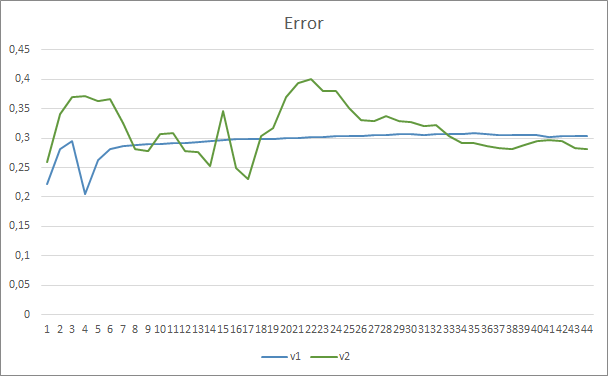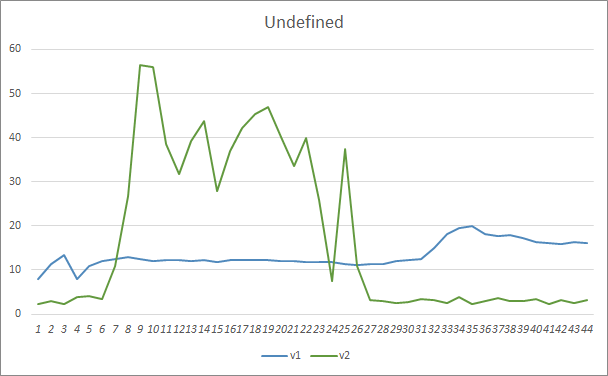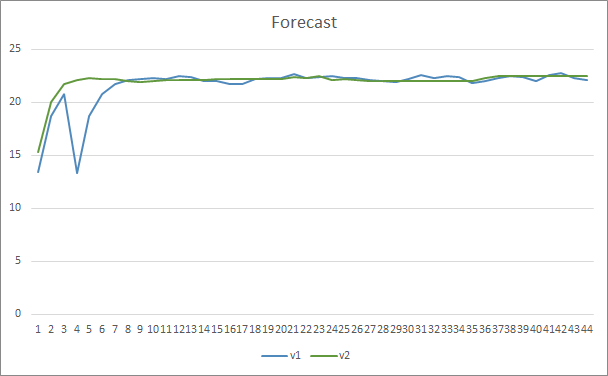### 本文中用到的程序

# 名称 类型 说明
1 Fractal_OCL_AttentionMLMH.mq5   智能交易系统 采用 GTP 架构的分类神经网络（输出层中有 3 个神经元）和 5 个关注层的智能交易系统
2 Fractal_OCL_AttentionMLMH_v2.mq5 智能交易系统   采用 GTP 架构的分类神经网络（输出层中有 3 个神经元）和 12 个关注层的智能交易系统
3 NeuroNet.mqh 类库 用于创建神经网络的类库
4 NeuroNet.cl 代码库 OpenCL 程序代码库
5 NN.chm HTML 帮助 经编译的 CHM 文件。

MQL5.zip (2306.29 KB)

#### 该作者的其他文章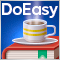DoEasy 函数库中的价格（第六十四部分）：市场深度，DOM 快照类和快照序列对象DoEasy 函数库中的价格（第六十三部分）：市场深度及其抽象请求类DoEasy 函数库中的价格（第六十五部分）：市场深度集合并操控 MQL5.com 信号的类DoEasy 函数库中的价格（第六十二部分）：实时更新即时报价序列，为操控市场深度做准备